# Solving Equations by Factoring Definition of Quadratic Equations

• Slides: 15Solving Equations by Factoring Definition of Quadratic Equations Zero-Factor Property Strategy for Solving Quadratics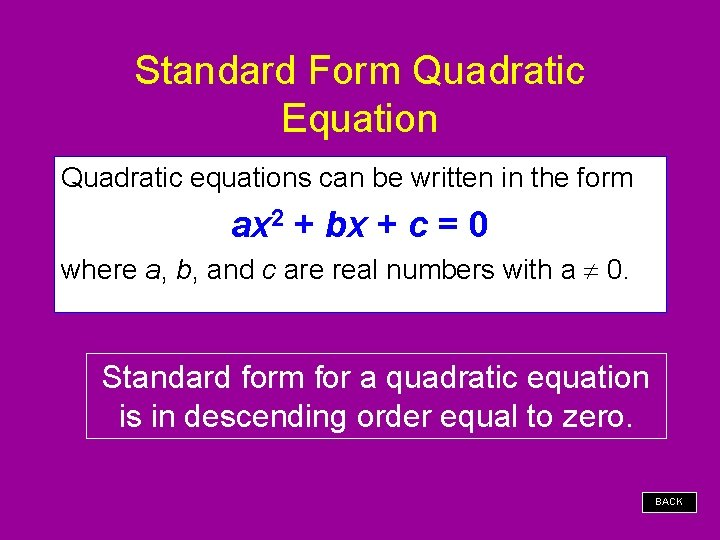Standard Form Quadratic Equation Quadratic equations can be written in the form ax 2 + bx + c = 0 where a, b, and c are real numbers with a 0. Standard form for a quadratic equation is in descending order equal to zero. BACKExamples of Quadratic Equations Standard Form BACK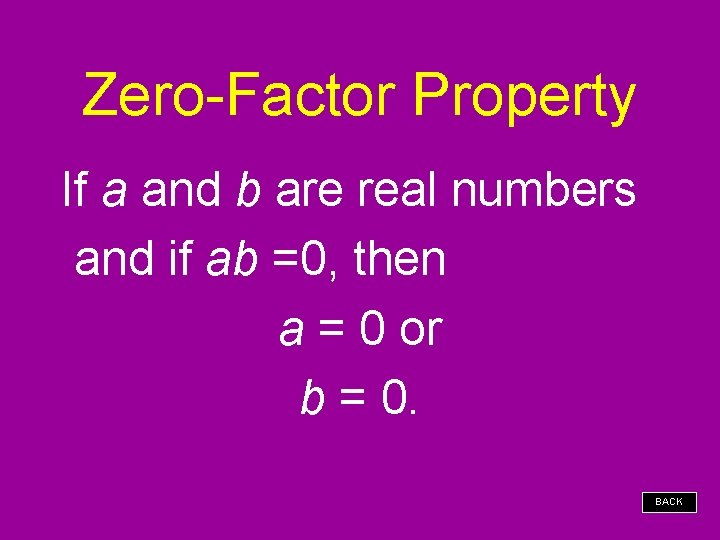Zero-Factor Property If a and b are real numbers and if ab =0, then a = 0 or b = 0. BACK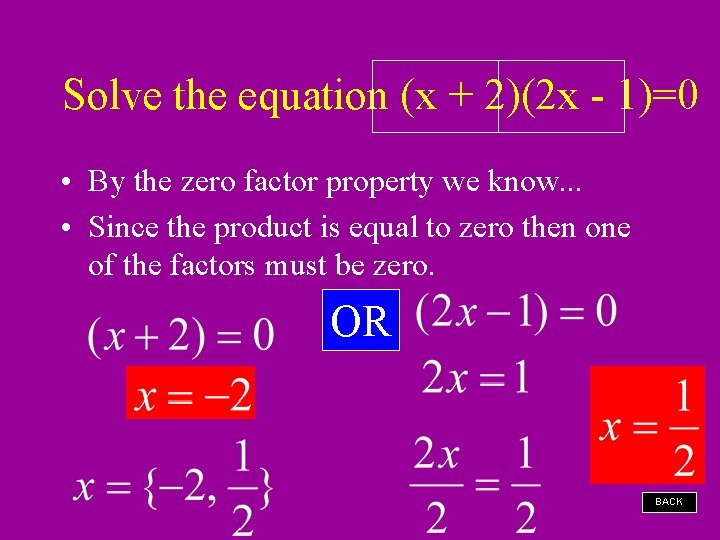Solve the equation (x + 2)(2 x - 1)=0 • By the zero factor property we know. . . • Since the product is equal to zero then one of the factors must be zero. OR BACKSolve the equation. Check your answers. OR Solution Set BACKSolve each equation. Check your answers. OR Solution Set BACK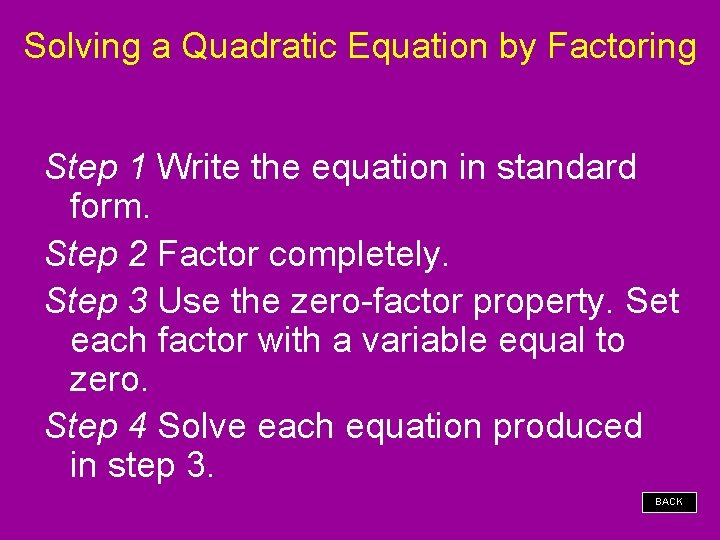Solving a Quadratic Equation by Factoring Step 1 Write the equation in standard form. Step 2 Factor completely. Step 3 Use the zero-factor property. Set each factor with a variable equal to zero. Step 4 Solve each equation produced in step 3. BACK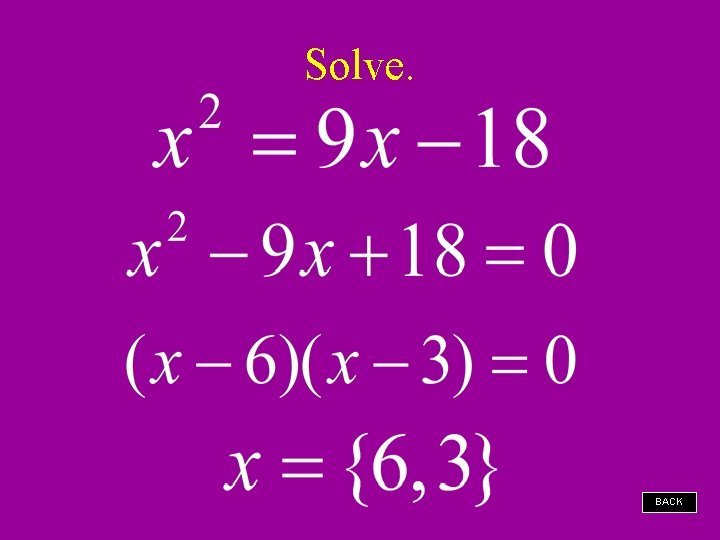Solve. BACKSolve. BACK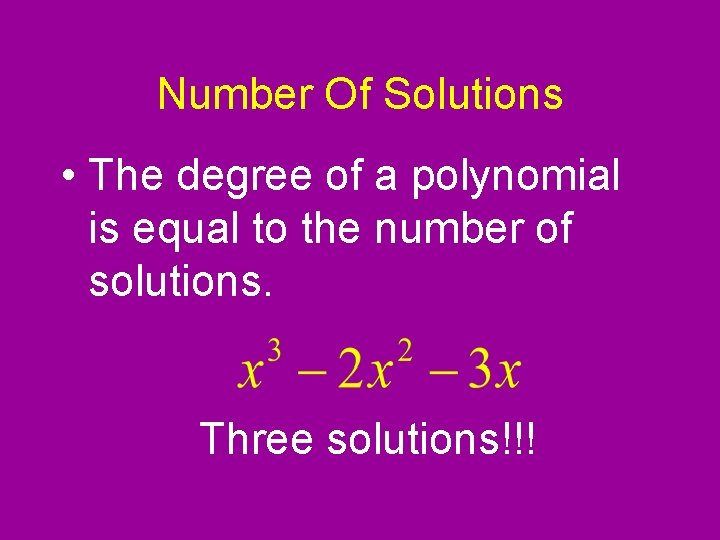Number Of Solutions • The degree of a polynomial is equal to the number of solutions. Three solutions!!!Example x (x + 1)(x – 3) = 0 Set each of the three factors equal to 0. x=0 x+1=0 x = -1 x– 3=0 x=3 Solve the resulting equations. Write the solution set. x = {0, -1, 3} BACKSolve the following equations. 1. x 2 – 25 = 0 2. x 2 + 7 x – 8 = 0 3. x 2 – 12 x + 36 = 0 4. c 2 – 8 c = 01. Get a value of zero on one side of the equation. 2. Factor the polynomial if possible. 3. Apply the zero product property by setting each factor equal to zero. 4. Solve for the variable.Solving Equations by Factoring Definition of Quadratic Equations Zero-Factor Property Strategy for Solving Quadratics